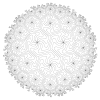Equations in One-Relator Groups and Semigroups September 3-7, 2018The focused research group Equations in One-Relator Groups and Semigroups will be held on 3-7 September 2018 in the new building of the International Centre for Mathematical Sciences (ICMS), Edinburgh. WHERE is ICMS ?. Topic This research group will focus on finding and understanding the solutions of equations in one-relator (semi)groups. Solving equations in infinite discrete (semi)groups is a topic at the wild frontier between soluble and insoluble, with far-reaching applications to algebra, logic, and theoretical computer science. Solving equations in groups goes much beyond the conjugacy problem, so will in general be insoluble. However, for free groups, Makanin and Razborov gave the first algorithm in the early 1980s, and since then the study of equations has extended to groups that are ‘close to free’ from the geometry or logic point of view, such as the right-angled Artin, or (relatively) hyperbolic ones. Problems and Aim. Our group will work on mapping out completely uncharted territory and bring together participants who have never interacted before in order to decide whether and when solving equations in one-relator groups is possible. We will start with Baumslag-Solitar groups, and then move on to more general one-relator amalgamated products and HNN-extensions. It is a celebrated result of Magnus that the word problem is soluble in one-relator groups, but it is still open whether the conjugacy problem is, so we must restrict ourselves to classes of groups where these basic problems are well understood. In case the satisfiability of general equations cannot be established, we will consider one-variable and quadratic equations, which are usually easier to tackle. We will also study one-relator (inverse) semigroups with soluble word problem.

Timetable

This meeting is supported by a Heilbronn Institute for Mathematical Research grant.

The local organiser for this meeting is Laura Ciobanu.